# Completing the square - example 3

Complete the square   2x^2-7x+6=0

NOTE:

The coefficient (or number) in front of the x^2 must be a one.

So divide both sides by 2

(2x^2)/2-(7x)/2+6/2=0/2

x^2-(7/2)x+3=0

Remember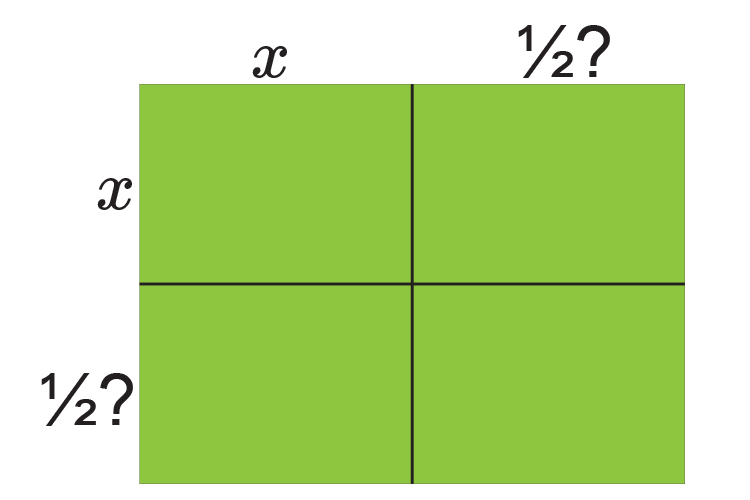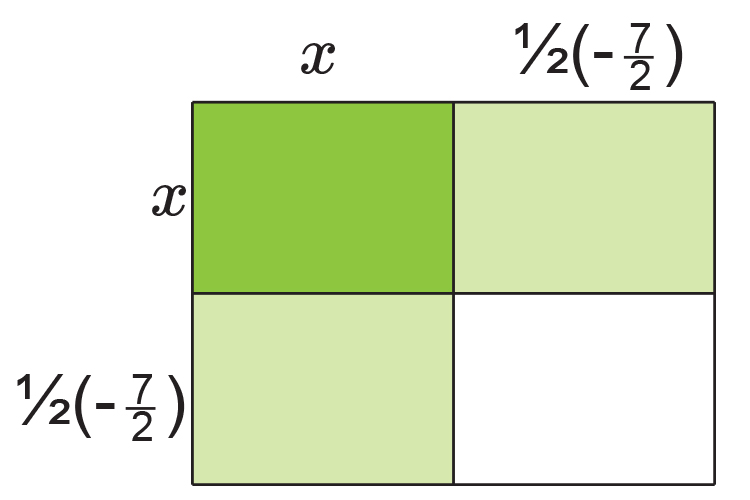Is the same as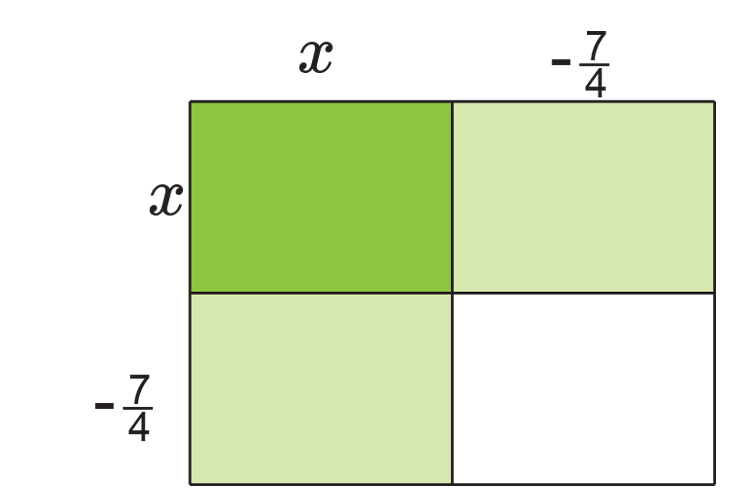Fill in the table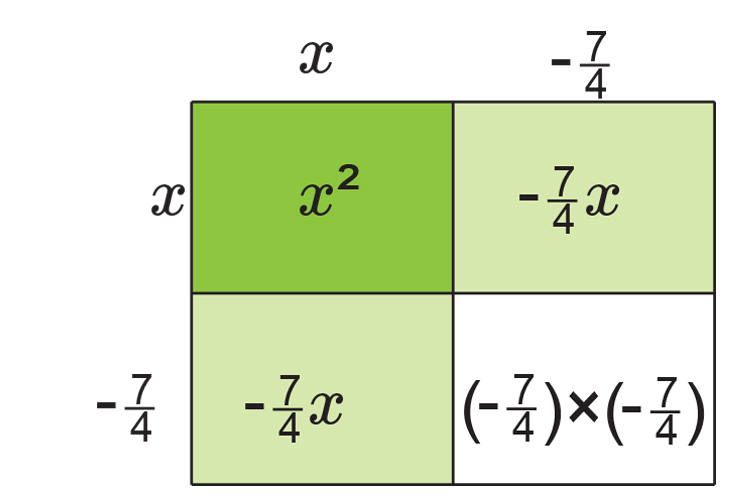Is the same as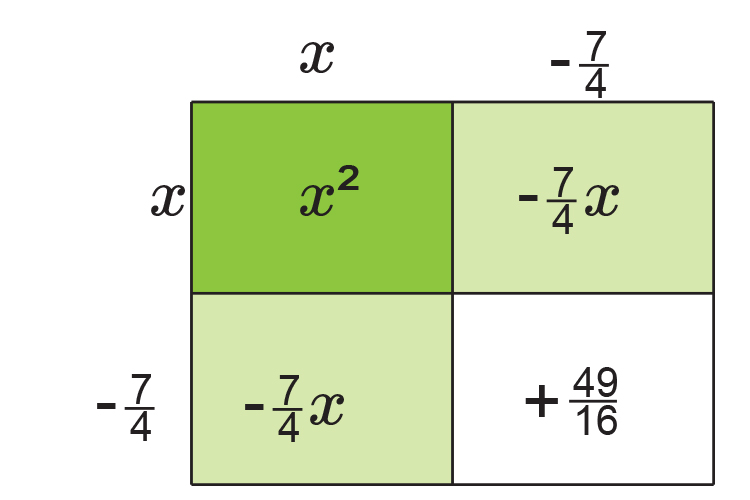NOTE:

This is the same as (x-7/4)^2

If you add up each area we get:

x^2-7/4x-7/4x+49/16

x^2-2times7/4x+49/16

x^2-7/2x+49/16

x^2-7/2x+3\1/16

Originally we had   x^2-7/2x+3=0

We now have         x^2-7/2x+3\1/16=0

Always plot this on a number line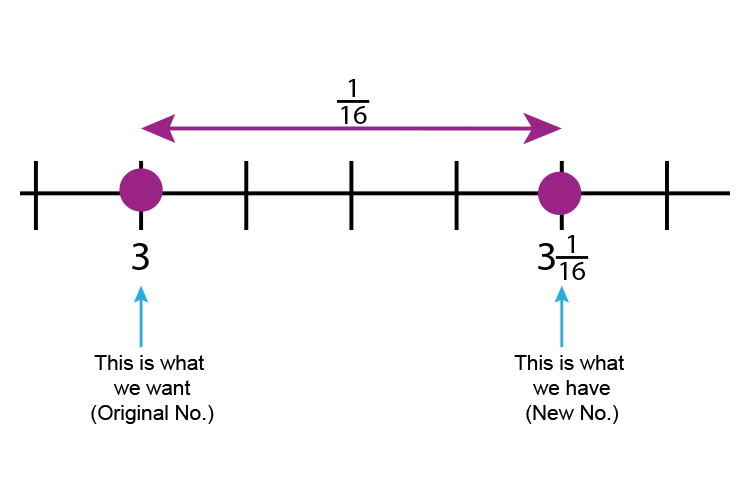Original -   New number

3-3\1/16=-1/16    (We need to -1/16  )

So                         x^2-7/2x+3=0

Is the same as

(x-7/4)^2-1/16=0

(x-7/4)^2=1/16

x-7/4=+-sqrt(1/16)

(Don't forget the root of anything can be + or -)

x-7/4=+-1/4

x=7/4+-1/4

x=7/4+1/4   or  x=7/4-1/4

x=8/4   or  6/4

x=2   or  1\1/2

### Now check

2x^2-7x+6=0

If x=2              2times2^2-7times2+6=0

8-14+6=0

If x=1\1/2         2times(3/2)^2-7times(3/2)+6=0

2times(9/4)-21/2+6=0

18/4-21/2+6=0

4\2/4-10\1/2+6=0

4\1/2-10\1/2+6=0   Which is correct

The roots of 2x^2-7x+6=0   are  x=2  or  x=1\1/2